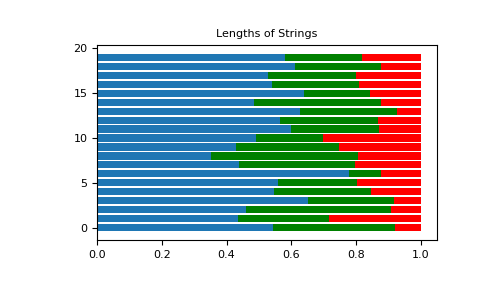#### Previous topic

numpy.random.Generator.chisquare

#### Next topic

numpy.random.Generator.exponential

# numpy.random.Generator.dirichlet¶

method

Generator.dirichlet(alpha, size=None)

Draw samples from the Dirichlet distribution.

Draw size samples of dimension k from a Dirichlet distribution. A Dirichlet-distributed random variable can be seen as a multivariate generalization of a Beta distribution. The Dirichlet distribution is a conjugate prior of a multinomial distribution in Bayesian inference.

Parameters
alphasequence of floats, length k

Parameter of the distribution (length k for sample of length k).

sizeint or tuple of ints, optional

Output shape. If the given shape is, e.g., (m, n), then m * n * k samples are drawn. Default is None, in which case a vector of length k is returned.

Returns
samplesndarray,

The drawn samples, of shape (size, k).

Raises
ValueError

If any value in alpha is less than or equal to zero

Notes

The Dirichlet distribution is a distribution over vectorsthat fulfil the conditionsand.

The probability density functionof a Dirichlet-distributed random vectoris proportional towhereis a vector containing the positive concentration parameters.

The method uses the following property for computation: letbe a random vector which has components that follow a standard gamma distribution, thenis Dirichlet-distributed

References

1

David McKay, “Information Theory, Inference and Learning Algorithms,” chapter 23, http://www.inference.org.uk/mackay/itila/

2

Wikipedia, “Dirichlet distribution”, https://en.wikipedia.org/wiki/Dirichlet_distribution

Examples

Taking an example cited in Wikipedia, this distribution can be used if one wanted to cut strings (each of initial length 1.0) into K pieces with different lengths, where each piece had, on average, a designated average length, but allowing some variation in the relative sizes of the pieces.

>>> s = np.random.default_rng().dirichlet((10, 5, 3), 20).transpose()

>>> import matplotlib.pyplot as plt
>>> plt.barh(range(20), s)
>>> plt.barh(range(20), s, left=s, color='g')
>>> plt.barh(range(20), s, left=s+s, color='r')
>>> plt.title("Lengths of Strings")